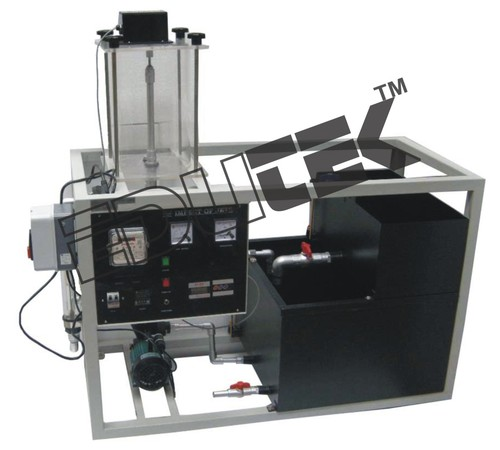0Impact of Jet of Water Apparatus
Product Specifications
• Mild Steel
Product Description

Impact of a Jet of Water

Descriptions of  Impact of a Jet

Impact of a Jet of Water The object of this experiment was to determine the force exerted by a jet of water on a stationary vane and compare the experimental results to the theoretical results.

Specification of Impact of a Jet

• To calculate the theoretical force a jet exerts on a surface.
• Assuming the impact velocity is the same as nozzle exit velocity.
• calculate the theoretical impact velocity.
• Using the theoretical equation for the impact force of a jet, calculate the impact force.
• Compare the theoretical and measured values.
• To determine the reaction force produced by the impact of jet of water of variety type of target vanes.